The TIMEDATA Procedure

Time Series Transformation

Four transformations are available for strictly positive series only. Let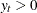be the original time series, and let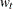be the transformed series. The transformations are defined as follows:

Log

is the logarithmic transformation.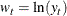Logistic

is the logistic transformation.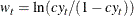where the scaling factoris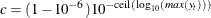and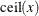is the smallest integer greater than or equal to x.

Square root

is the square root transformation.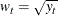Box Cox

is the Box-Cox transformation.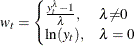More complex time series transformations can be performed by using the SAS/ETS EXPAND procedure.